## Hardcover edition of Geometric Algebra for Electrical Engineers.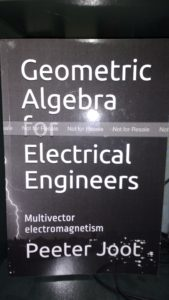I was invited to Kindle Direct Publishing‘s hardcover beta program, and have made my geometric algebra book available in black and white hardcover.

As always, the PDF, leanpub edition, and latex sources are also available.

I thought that it was too confusing to have color and black-and-white editions of the book (color has a significantly higher printing cost), so I have unpublished the color editions of the book (softcover, and hardcover). There is one copy of the color edition left, and once that is sold, it will show as out of print.

## A better 3D generalization of the Mandelbrot set.

I’ve been exploring 3D generalizations of the Mandelbrot set:

The iterative equation for the Mandelbrot set can be written in vector form () as:
\begin{equation}
\begin{aligned}
\Bz
&\rightarrow
\Bz \Be_1 \Bz + \Bc \\
&=
\Bz \lr{ \Be_1 \cdot \Bz }
+
\Bz \cdot \lr{ \Be_1 \wedge \Bz }
+ \Bc \\
&=
2 \Bz \lr{ \Be_1 \cdot \Bz }

\Bz^2\, \Be_1
+ \Bc
\end{aligned}
\end{equation}
Plotting this in 3D was an interesting challenge, but showed that the Mandelbrot set expressed above has rotational symmetry about the x-axis, which is kind of boring.

If all we require for a 3D fractal is to iterate a vector equation that is (presumably) at least quadratic, then we have lots of options. Here’s the first one that comes to mind:
\begin{equation}
\begin{aligned}
\Bz
&\rightarrow
\gpgradeone{ \Ba \Bz \Bb \Bz \Bc } + \Bd \\
&=
\lr{ \Ba \cdot \Bz } \lr{ \Bz \cross \lr{ \Bc \cross \Bz } }
+
\lr{ \Ba \cross \Bz } \lr{ \Bz \cdot \lr{ \Bc \cross \Bz } }
+ \Bd
.
\end{aligned}
\end{equation}
where we iterate starting, as usual with $$\Bz = 0$$ where $$\Bd$$ is the point of interest to test for inclusion in the set. I tried this with
\begin{equation}\label{eqn:mandel3:n}
\begin{aligned}
\Ba &= (1,1,1) \\
\Bb &= (1,0,0) \\
\Bc &= (1,-1,0).
\end{aligned}
\end{equation}
Here are some slice plots at various values of z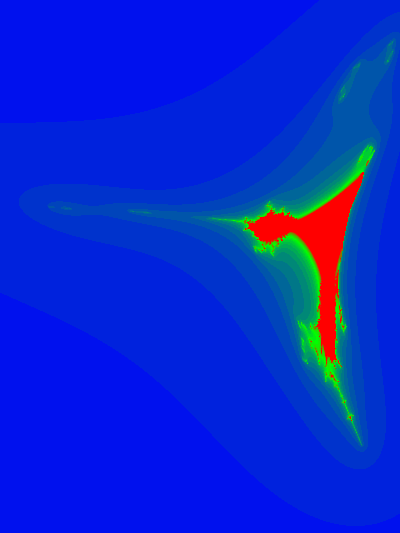and an animation of the slices with respect to the z-axis:Here are a couple snapshots from a 3D Paraview rendering of a netCDF dataset of all the escape time values

Data collection and image creation used commit b042acf6ab7a5ba09865490b3f1fedaf0bd6e773 from my Mandelbrot generalization experimentation repository.

# References

 L. Dorst, D. Fontijne, and S. Mann. Geometric Algebra for Computer Science. Morgan Kaufmann, San Francisco, 2007.

## Some slices.

As followup to:

here is a bit more experimentation with Paraview slice filtering. This time I saved all the point data with the escape time counts, and rendered it with a few different contours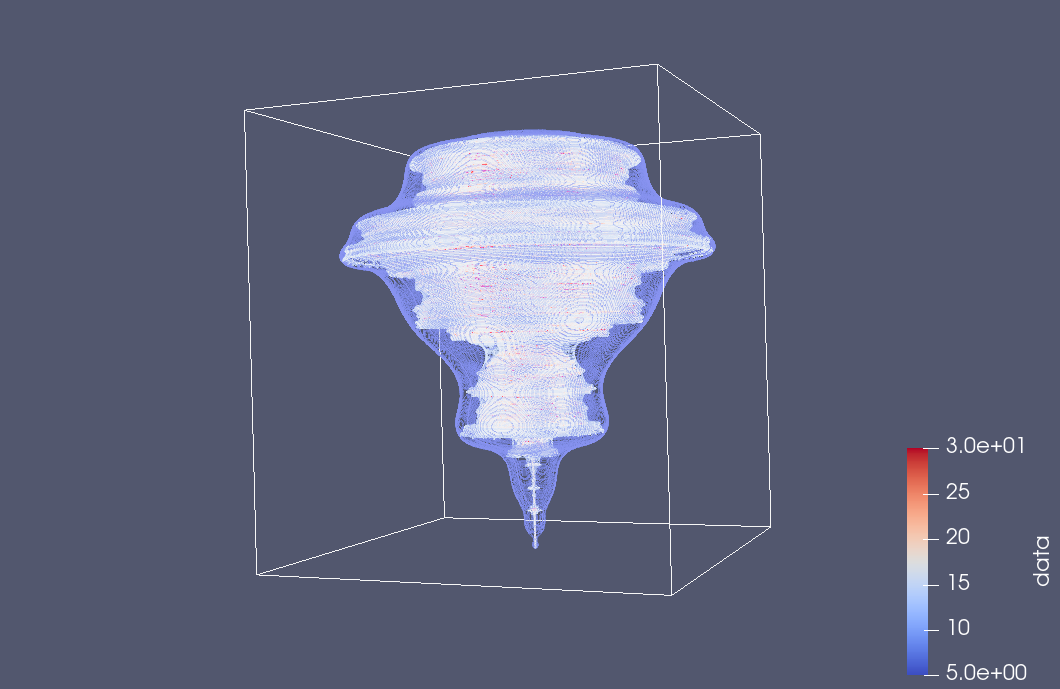The default slice filter places the plane in the x-y orientation:but we can also tilt it in the Paraview render UI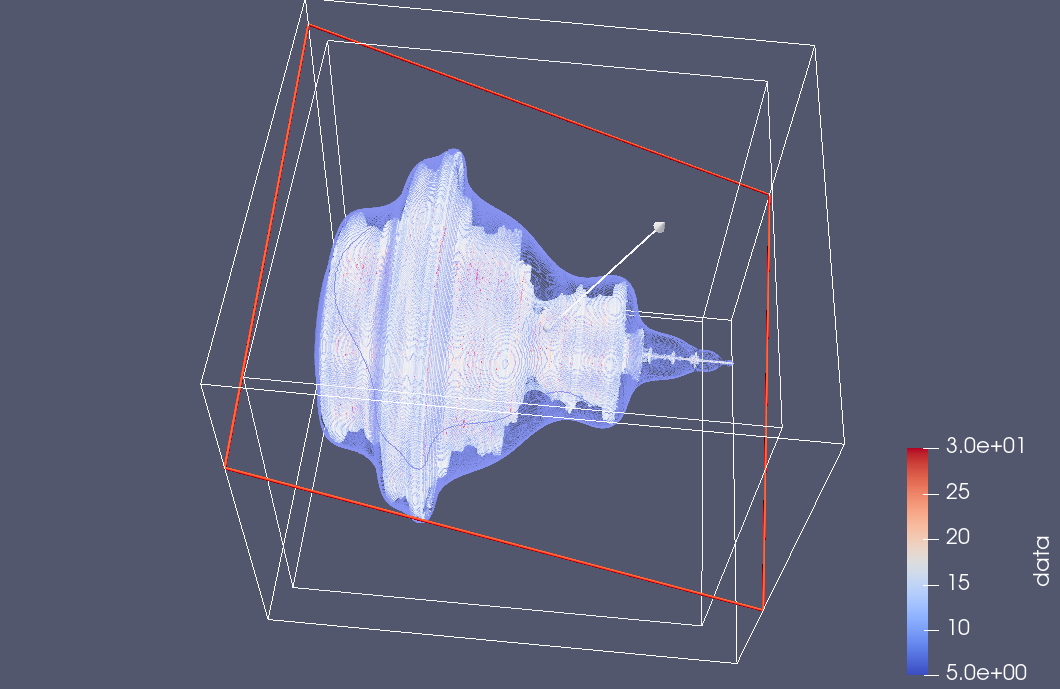and suppress the contour view to see just the slice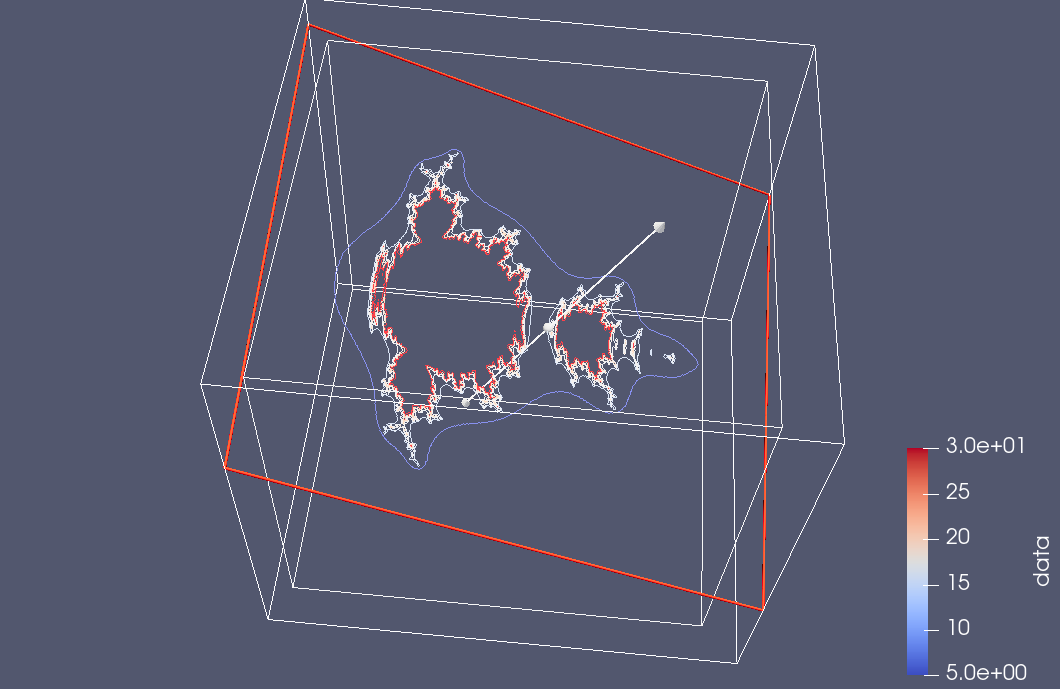As a very GUI challenged user, I don’t find the interface particularly intuitive, but have at least figured out this one particular slicing task, which is kind of cool.  It’s impressive that the UI can drive interesting computational tasks without having to regenerate or reload any of the raw data itself.  This time I was using the MacOSX Paraview client, which is nicer looking than the Windows version, but has some weird glitches in the file dialogues.

## Analysis.

The graphing play above shows some apparent rotational symmetry our vector equivalent to the Mandelbrot equation
\begin{equation}
\Bx \rightarrow \Bx \Be_1 \Bx + \Bc.
\end{equation}
It was not clear to me if this symmetry existed, as there were artifacts in the plots that made it appear that there was irregularity. However, some thought shows that this irregularity is strictly due to sampling error, and perhaps also due to limitations in the plotting software, as such an uneven surface is probably tricky to deal with.

To see this, here are the first few iterations of the Mandlebrot sequence for an arbitary starting vector $$\Bc$$.
\begin{equation}
\begin{aligned}
\Bx_0 &= \Bc \\
\Bx_1 &= \Bc \Be_1 \Bc + \Bc \\
\Bx_2 &= \lr{ \Bc \Be_1 \Bc + \Bc } \Be_1 \lr{ \Bc \Be_1 \Bc + \Bc } + \Bc \Be_1 \Bc + \Bc.
\end{aligned}
\end{equation}

Now, what happens when we rotate the starting vector $$\Bc$$ in the $$y-z$$ plane. The rotor for such a rotation is
\begin{equation}
R = \exp\lr{ e_{23} \theta/2 },
\end{equation}
where
\begin{equation}
\Bc \rightarrow R \Bc \tilde{R}.
\end{equation}
Observe that if $$\Bc$$ is parallel to the x-axis, then this rotation leaves the starting point invariant, as $$\Be_1$$ commutes with $$R$$. That is
\begin{equation}
R \Be_1 \tilde{R} =
\Be_1 R \tilde{R} = \Be_1.
\end{equation}
Let $$\Bc’ = R \Bc \tilde{R}$$, so that
\begin{equation}
\Bx_0′ = R \Bc \tilde{R} = R \Bx_0 \tilde{R} .
\end{equation}
\begin{equation}
\begin{aligned}
\Bx_1′
&= R \Bc \tilde{R} \Be_1 R \Bc \tilde{R} + R \Bc \tilde{R} \\
&= R \Bc \Be_1 \Bc \tilde{R} + R \Bc \tilde{R} \\
&= R \lr{ \Bc \Be_1 \Bc R + \Bc } \tilde{R} \\
&= R \Bx_1 \tilde{R}.
\end{aligned}
\end{equation}
\begin{equation}\label{eqn:m2:n}
\begin{aligned}
\Bx_2′
&= \Bx_1′ \Be_1 \Bx_1′ + \Bc’ \\
&= R \Bx_1 \tilde{R} \Be_1 R \Bx_1 \tilde{R} + R \Bc \tilde{R} \\
&= R \Bx_1 \Be_1 \Bx_1 \tilde{R} + R \Bc \tilde{R} \\
&= R \lr{ \Bx_1 \Be_1 \Bx_1 + \Bc } \tilde{R} \\
&= R \Bx_2 \tilde{R}.
\end{aligned}
\end{equation}

The pattern is clear. If we rotate the starting point in the y-z plane, iterating the Mandelbrot sequence results in precisely the same rotation of the x-y plane Mandelbrot sequence. So the apparent rotational symmetry in the 3D iteration of the Mandelbrot vector equation is exactly that. This is an unfortunately boring 3D fractal. All of the interesting fractal nature occurs in the 2D plane, and the rest is just a consequence of rotating that image around the x-axis. We get some interesting fractal artifacts if we slice the rotated Mandelbrot image.

## Some 3D renderings of the Mandelbrot set.

As mentioned previously, using geometric algebra we can convert the iterative equation for the Mandelbrot set from complex number form
\begin{equation}
z \rightarrow z^2 + c,
\end{equation}
to an equivalent vector form
\begin{equation}
\mathbf{x} \rightarrow \mathbf{x} \mathbf{e} \mathbf{x} + \mathbf{c},
\end{equation}
where $$\mathbf{e}$$ represents the x-axis (say). Geometrically, each iteration takes $$\mathbf{e}$$ and reflects it about the direction of $$\mathbf{x}$$, then scales that by $$\mathbf{x}^2$$ and adds $$\mathbf{c}$$.

To get the usual 2D Mandelbrot set, one iterates with vectors that lie only in the x-y plane, but we can treat the Mandelbrot set as a 3D solid if we remove the x-y plane restriction.

Last time I animated slices of the 3D set, but after a whole lot of messing around I managed to save the data for all the interior points of the 3D set in netCDF format, and render the solid using Paraview. Paraview has tons of filters available, and experimenting with them is pretty time consuming, but here are some initial screenshots of the 3D Mandelbrot set: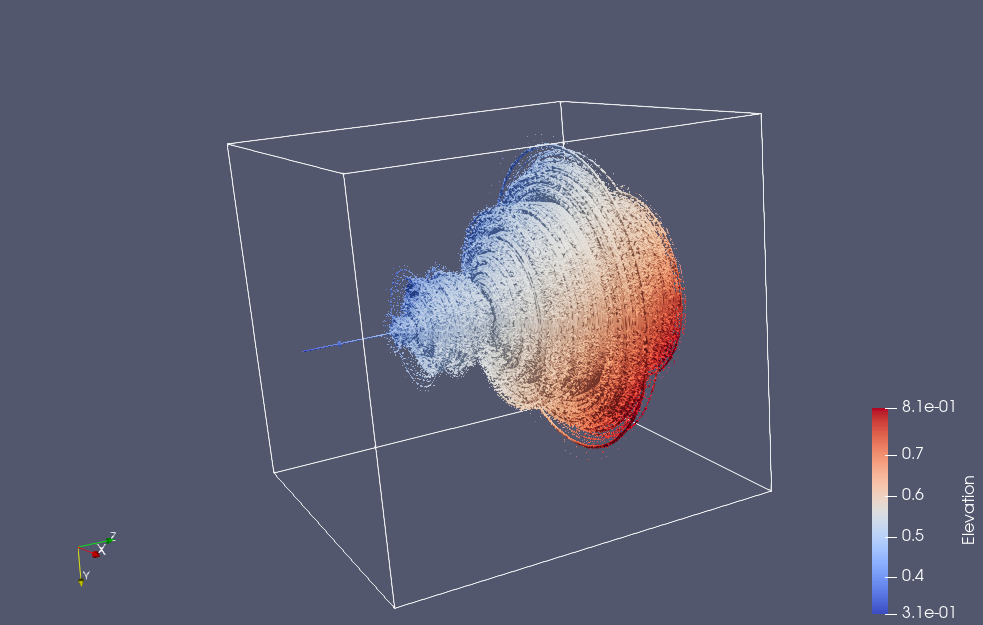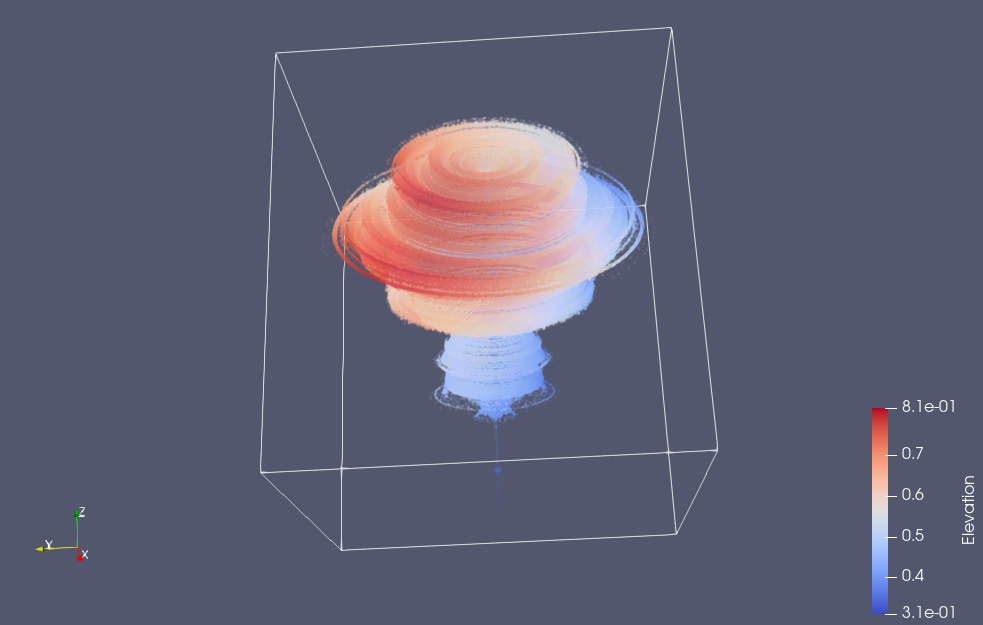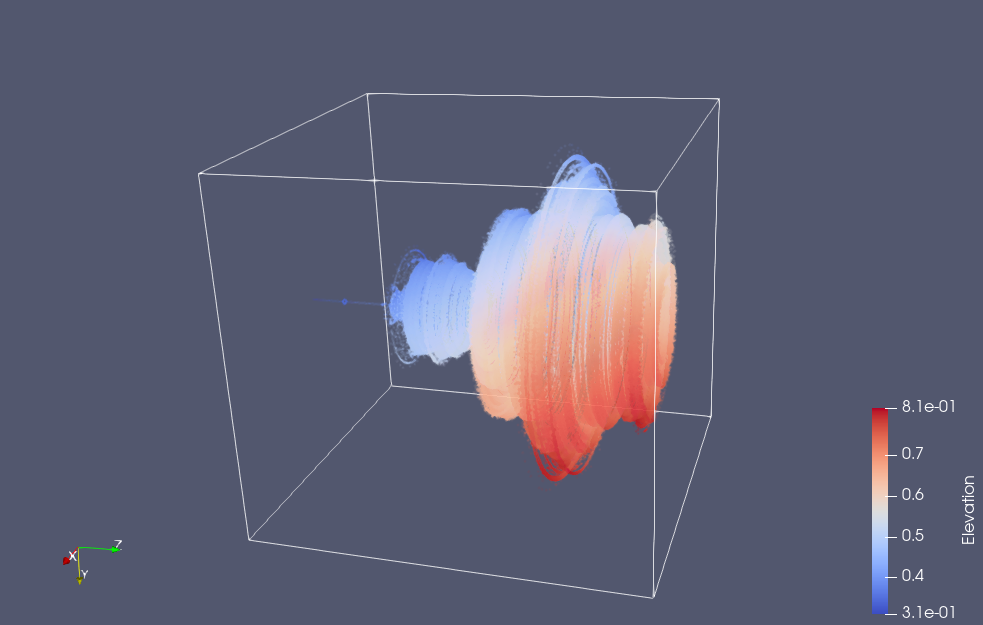It’s interesting that much of the characteristic detail of the Mandelbrot set is not visible in the 3D volume, but if we slice that volume, you can then you can see it again.  Here’s a slice taken close to the z=0 plane (but far enough that the “CN tower” portion of the set is not visible)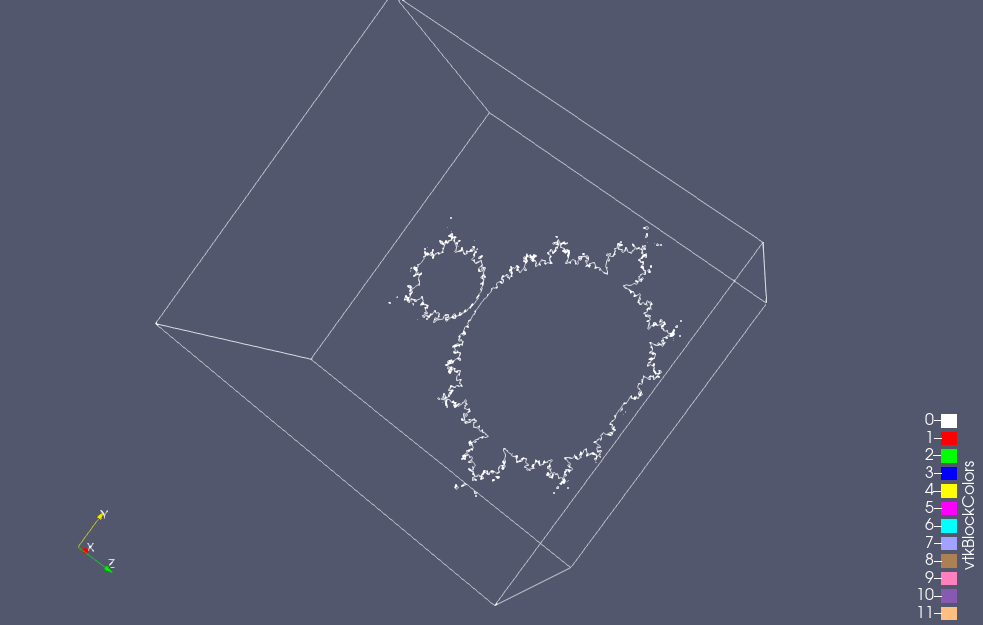You can also see some of that detail if the opacity of the rendering is turned way down:If you look carefully at the images above, you’ll see that the axis labels are wrong.  I think that I’ve screwed up one of the stride related parameters to my putVar call, and I end up with x+z transposed in the axes labels when visualized.

## Visualizing the 3D Mandelbrot set.

February 1, 2021 C/C++ development and debugging.

In “Geometric Algebra for Computer Science” is a fractal problem based on a vectorization of the Mandelbrot equation, which allows for generalization to $$N$$ dimensions.

I finally got around to trying the 3D variation of this problem.  Recall that the Mandlebrot set is a visualization of iteration of the following complex number equation:
\begin{equation}
z \rightarrow z^2 + c,
\end{equation}
where the idea is that $$z$$ starts as the constant $$c$$, and if this sequence converges to zero, then the point $$c$$ is in the set.

The idea in the problem is that this equation can be cast as a vector equation, instead of a complex number equation. All we have to do is set $$z = \Be_1 \Bx$$, where $$\Be_1$$ is the x-axis unit vector, and $$\Bx$$ is an $$\mathbb{R}^2$$ vector. Expanding in coordinates, with $$\Bx = \Be_1 x + \Be_2 y$$, we have
\begin{equation}
z
= \Be_1 \lr{ \Be_1 x + \Be_2 y }
= x + \Be_1 \Be_2 y,
\end{equation}
but since the bivector $$\Be_1 \Be_2$$ squares to $$-1$$, we can represent complex numbers as even grade multivectors. Making the same substitution in the Mandlebrot equation, we have
\begin{equation}
\Be_1 \Bx \rightarrow \Be_1 \Bx \Be_1 \Bx + \Be_1 \Bc,
\end{equation}
or
\begin{equation}
\Bx \rightarrow \Bx \Be_1 \Bx + \Bc.
\end{equation}
Viola! This is a vector version of the Mandlebrot equation, and we can use it in 2 or 3 or N dimensions, as desired.  Observe that despite all the vector products above, the result is still a vector since $$\Bx \Be_1 \Bx$$ is the geometric algebra form of a reflection of $$\Bx$$ about the x-axis.

The problem with generalizing this from 2D is really one of visualization. How can we visualize a 3D Mandelbrot set? One idea I had was to use ray tracing, so that only the points on the surface from the desired viewpoint need be evaluated. I don’t think I’ve ever written a ray tracer, but I thought that there has got to be a quick and dirty way to do this.  Also, figuring out how to make a ray tracer interact with an irregular surface like this is probably non trivial!

What I did instead, was a brute force evaluation of all the points in the upper half plane in around the origin, one slice of the set at a time. Here’s the result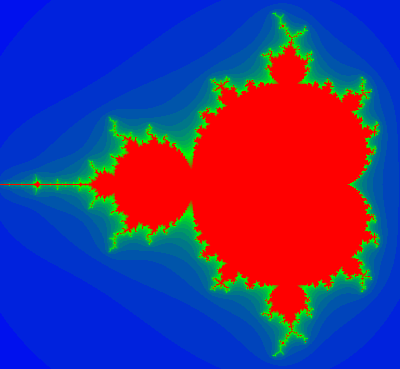Code for the visualization can be found in github. I’ve used Pauli matrices to do the evaluation, which is actually pretty quick (but slower than plain std::complex< double> evaluation), and the C++ ImageMagick API to save individual png files for the slices. There are better coloring schemes for the Mandelbrot set, and if I scrounge up some more time, I may try one of those instead.